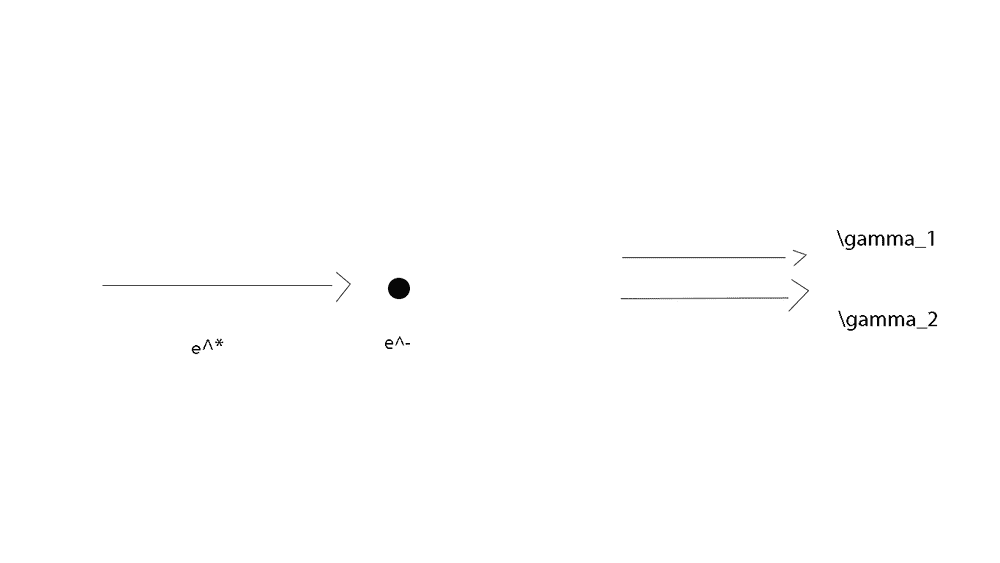# Annihilation: calculation of photon energies

• Frostman
In summary, the conversation discusses the conservation of quadri-momenta in the problem of two particles annihilating and producing two photons. The speaker considers the two photons as a single particle with energy equal to twice the energy of a single photon. However, the other person points out that this assumption is invalid as the two photons must have different energies and be moving in opposite directions. The conversation then moves on to discuss finding the energies in the reference system integral with the incident particle, and the speaker suggests using Lorentz transformations to do so.f

#### Frostman

Homework Statement
An antiparticle of mass ##m## and energy ##E## hits a stationary particle in the laboratory and the system annihilates itself producing two photons, which are directed along the direction of the motion of the incident antiparticle.
1 - Calculate the energies of the two photons in the laboratory's reference system.
2 - Calculate the energies in the reference system integral with the incident particle, explicitly carrying out the Lorentz transformation. Discuss the result obtained.
Relevant Equations
I set up this problem this way:

##p_a^{\mu}=(E, \sqrt{E^2-m^2}, 0, 0)##
##p_b^{\mu}=(m, 0, 0, 0)##
##p_c^{\mu}=(2E_\gamma, 2E_\gamma, 0, 0)##

I have chosen to consider the two photons as a single particle of energy equal to ##2E_\gamma##. At this point I applied conservation of the quadri-momenta:

##E+m=2E_\gamma##
##\sqrt{E^2-m^2}=2E_\gamma##

From the first equation I find the first point: ##E_\gamma=\frac {E+m}2##. But if I put this in the second equation I don't have the equality. Why?
I'm supposing that the particle ##a## is a ##e^+## and particle ##b## is an ##e^-##, because the statement said that the system annihilates itself.

I have chosen to consider the two photons as a single particle of energy equal to ##2E_\gamma##.
How can this possibly be justified?

How can this possibly be justified?
Because they are the same particle. I could write:
##p_{\gamma 1}^\mu=(E_\gamma, E_\gamma, 0,0)##
##p_{\gamma 2}^\mu=(E_\gamma, E_\gamma, 0,0)##

•PeroK
Because they are the same particle. I could write:
##p_{\gamma 1}^\mu=(E_\gamma, E_\gamma, 0,0)##
##p_{\gamma 2}^\mu=(E_\gamma, E_\gamma, 0,0)##
This is nonsense. 1) They must be moving in opposite directions and 2) They must have different energies.

This is nonsense. 1) They must be moving in opposite directions and 2) They must have different energies.
For
...which are directed along the direction of the motion of the incident antiparticle...
I was supposing this situation in LAB-frame:So then, they have the same direction, but opposite verse and different module?

For

I was supposing this situation in LAB-frame:
View attachment 276393
So then, they have the same direction, but opposite verse and different module?
They must be in opposite directions in all IRF's, as they must be in opposite directions in the CM frame.

•Frostman
I understand. I had to start thinking from the CM how the situation should be and then think about how the situation changed in the different IRF's.
In this case so I should start from:

##p_a^{\mu}=(E, \sqrt{E^2-m^2}, 0, 0)##
##p_b^{\mu}=(m, 0, 0, 0)##
##p_c^{\mu}=(E_{\gamma 1}, E_{\gamma 1}, 0, 0)##
##p_d^{\mu}=(E_{\gamma 2}, - E_{\gamma 2}, 0, 0)##

Considering the conservation of the quadrimoment, moving the term ##p_c^\mu## to the first member and squaring the members I obtain:

##p_a^{\mu}+p_b^{\mu}=p_c^{\mu}+p_d^{\mu}##
##(p_a^{\mu}+p_b^{\mu}-p_c^{\mu})^2=(p_d^{\mu})^2##
##p_a^2+p_b^2+p_c^2+2p_a^\mu p_{\mu b}-2p_a^\mu p_{\mu c}-2p_b^\mu p_{\mu c}=0##
##m^2+m^2+2Em-2(EE_{\gamma 1}-E_{\gamma1}\sqrt{E^2-m^2})-2mE_{\gamma 1}##

##E_{\gamma 1}=\frac{m(E+m)}{E+m-\sqrt{E^2-m^2}}=\frac{m}{1-\sqrt{\frac{E-m}{E+m}}}##

Similarly for ##E_{\gamma 2}##.

I understand. I had to start thinking from the CM how the situation should be and then think about how the situation changed in the different IRF's.
In this case so I should start from:

##p_a^{\mu}=(E, \sqrt{E^2-m^2}, 0, 0)##
##p_b^{\mu}=(m, 0, 0, 0)##
##p_c^{\mu}=(E_{\gamma 1}, E_{\gamma 1}, 0, 0)##
##p_d^{\mu}=(E_{\gamma 2}, - E_{\gamma 2}, 0, 0)##

Considering the conservation of the quadrimoment, moving the term ##p_c^\mu## to the first member and squaring the members I obtain:

##p_a^{\mu}+p_b^{\mu}=p_c^{\mu}+p_d^{\mu}##
##(p_a^{\mu}+p_b^{\mu}-p_c^{\mu})^2=(p_d^{\mu})^2##
##p_a^2+p_b^2+p_c^2+2p_a^\mu p_{\mu b}-2p_a^\mu p_{\mu c}-2p_b^\mu p_{\mu c}=0##
##m^2+m^2+2Em-2(EE_{\gamma 1}-E_{\gamma1}\sqrt{E^2-m^2})-2mE_{\gamma 1}##

##E_{\gamma 1}=\frac{m(E+m)}{E+m+\sqrt{E^2-m^2}}=\frac{m}{1+\sqrt{\frac{E-m}{E+m}}}##

Similarly for ##E_{\gamma 2}##.
I can't follow that at all, but it's definitely wrong.

Why not use conservation of energy and conservation of momentum?

I can't follow that at all, but it's definitely wrong.

Why not use conservation of energy and conservation of momentum?
Yes, I understand that I have to relax on the weekend or I shoot nonsense

##E_{\gamma 1} = \frac{E+m+\sqrt{E^2-m^2}}{2}##
##E_{\gamma 2} = \frac{E+m-\sqrt{E^2-m^2}}{2}##

•PeroK
2 - Calculate the energies in the reference system integral with the incident particle, explicitly carrying out the Lorentz transformation. Discuss the result obtained.
I suppose now to start from ##p_c^\mu## and ##p_d^\mu## and find ##p_c^{\mu'}## and ##p_d^{\mu'}## using Lorentz transformation for energy and momentum with ##\gamma=\frac E m## and ##v=\frac{\sqrt{E^2-m^2}}{E}##, or I have to find a different way?

I suppose now to start from ##p_c^\mu## and ##p_d^\mu## and find ##p_c^{\mu'}## and ##p_d^{\mu'}## using Lorentz transformation for energy and momentum with ##\gamma=\frac E m## and ##v=\frac{\sqrt{E^2-m^2}}{E}##, or I have to find a different way?
The question asks you to do the LT explicitly. So, I guess you have to do it that way.

Homework Statement:: An antiparticle of mass ##m## and energy ##E## hits a stationary particle in the laboratory and the system annihilates itself producing two photons
The stationary particle also has rest mass m (e.g. if we are dealing with a positron and an electron). So in the Lab' frame, you will want to ensure the sum of the two photon energies is E+2m, not E+m ((taking c=1).

•PeroK
The stationary particle also has rest mass m (e.g. if we are dealing with a positron and an electron). So in the Lab' frame, you will want to ensure the sum of the two photon energies is E+2m, not E+m ((taking c=1).
##E## is energy, not Kinetic Energy.

•Steve4Physics
Thanks. I misinterpreted that.